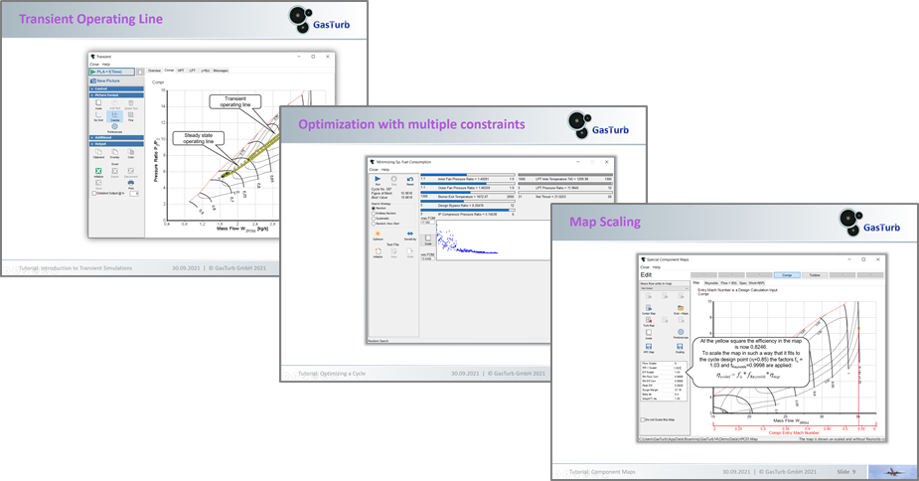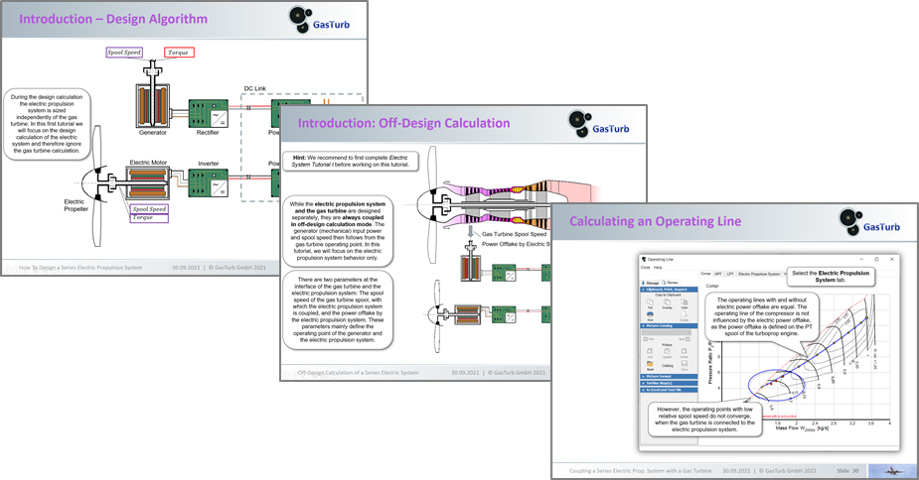GasTurb makes simulation tasks easy. The tutorials on this site give an overview of GasTurb's functionalities and provide a basis for applying the software to the gas turbine design process. The tutorials are designed for both newcomers and advanced users.

## Introduction to GasTurb

This three-part tutorial series provides an introduction to Gasturb's user interface and its most frequently used features.

Tutorial I: How to calculate a Gas Turbine cycle

• GasTurb Main Window
• Design Point Calculation
• Formula Editor
• h-s-Diagrams
• Iterations

Tutorial II: Design Parametric Study

• Conducting Parametric Studies
• Contours & Design Limits
• Parametric Studies with Iterations

Tutorial III: Introduction to Off-Design Simulations

• Off-Design Point Calculation
• Usage of Component Maps
• Operating Lines
• Handling Bleeds

The following PowerPoint tutorials deal with more advanced topics:

• Component Maps
• Optimizing a Cycle
• Preliminary Engine Design
• Control System Simulation
• Test Analysis
• Use of Unscaled Maps
• Introduction to Transient Simulations

Size: 20.6 MB
Type: ZIP## Electric Systems

These three PowerPoint tutorials describe the design and off-design calculation of hybrid-electric propulsion systems in GasTurb:

• Design of a Series Electric Propulsion System
• Off-Design Calculation
• Coupling with a Gas Turbine